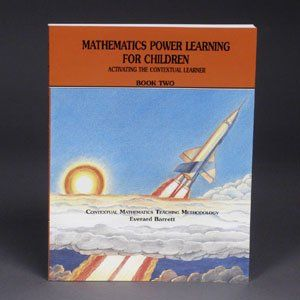Making You An Instant Master Teacher
•# Mathematics Power Learning Book 2 (3rd-5th)

\$25.00

For 3rd through 5th graders and remediation of older learners. Original and effective methods for thorough understanding and mastery of multiplication/division facts, multiplication, long division, fractional equivalence, addition/subtraction of fractions and mixed numbers. Your learners should complete Book II in 10 months or less.

Additional item needed: Power Learning Workbook 2

SAMPLE LESSON

TELLING THE TRUTH ABOUT LONG DIVISION

Objective: The learner will do long division examples with dividends up to six digits and divisors up to three digits.

Write the following problem on the board:

Your principal has \$275 and wishes to buy some workbooks for her school
How many can she buy if the cost of each book is \$4?

Have the learners read the problem.
Now write the following on the board:Tell learners that the number under the line is called the dividend (have them say "dividend"), and the number on the left is called the divisor (have them say "divisor").

Have them name each place value in the dividend.
Learners respond, "Ones, tens, hundreds."

Tell learners that there is a question for each value in the dividend and they must know how to ask each one.

Insist that each learner pretends to be the principal, as the questions are being asked.

The questions are listed below:

1. Hundreds' place: "Can I buy one hundred books?"
2. Tens' place: "Can I buy ten books?"
3. One's place: "Can I buy one book?"

Have learners practice asking the above questions to the level of facility.

Now return to the first question: "Can I buy one hundred books?"

Elicit from the learners that the cost of one hundred books is \$400. This is more than the amount of money (\$275) available. Hence no learner can buy one hundred books.

The board (and learners') work now looks like this:400

Insist that learners write 400 to the right of the division as it appears above.

The little "x" above the two in the hundreds' place means that each learner buys "zero hundreds" of books, since one hundred books cost too much. It also means each learner cannot buy any books in the hundreds' place.

Since each learner cannot buy 100 books, we move to the tens' place and ask, "Can I buy ten books?"

Elicit from learners that the cost of ten books is \$40. This is less than the amount of money (\$275) available. Hence each learner can buy 10 books.

The board (and learners') work now looks like this:40040

Now consider the digits: 0,1, 2, 3, 4, 5, 6, 7, 8, 9.
Explain to learners that in the tens' place, these digits represent 0 tens, 1 ten, 2 tens, 3 tens, and so on, up to 9 tens.

Tell them that since they can buy 10 books each, perhaps they can buy 20, or 30, or 40, and so on, up to 90 books each.
So the question is this: What are the maximum tens of books each learner can buy?

To estimate the maximum tens of books, we must use the digit in the divisor, 4, and the first digit, 2, in the dividend 275, and ask the question, "Four into two?"

Since four does not "go into" two, we must use the digit in the divisor, 4, and the first two digits, 27, in the dividend, and ask the question, "Four into twenty-seven?"

Tell learners that, in fact, we are really asking the question, "Four into two hundred seventy?"
The answer to "four into twenty-seven" is six (4 into 270 is approximately 60).

Have learners understand that this "six" means that from among the choices of 10 through 90 books, they must see if they can buy 60 books.

Have them see, by multiplication, that the cost of 60 books is \$240.
This is less than \$275; so the maximum tens of books which can be bought is six (which means 60 books).

The board work now looks like this:The board work shows learners that after 60 books have been bought, \$35 are left.

Ask, "Can you buy more books out of the remaining \$35?"

Elicit from learners that they must now move over to the ones' place and ask, "Can I buy one book?" Since one book costs \$4, they can.

Now let us return to the digits 0,1, 2, 3, 4, 5, 6, 7, 8, 9.

Explain to learners that in the ones' place, these digits represent zero, one, two, three, and so on, up to nine.

Tell them that since they can buy one book each, perhaps they can buy 2, or 3, or 4, and so on, up to 9 books each.
So the question is this: What are the maximum ones of books each learner can buy?

To estimate the maximum ones of books, we must use the digit in the divisor, 4, and the first digit, 3, in 35 and ask the question, "Four into three?"

Since four does not go into three, we must use the digit, 4, and the two digits, 35, and ask the question, "Four into thirty-five?"
The answer to the question is eight.

Have the learners agree that since we are in the ones' place, the "eight" means that each learner must try to buy eight books.

Have them see, by multiplication, that the cost of 8 books is \$32.
This is less than \$35, and the maximum ones of books which can be bought is 8 (which means 8 books).

The board work finally looks like this:Ask, "Can you buy any more books?" The board work shows that 68 books have been bought and \$3 are left over.